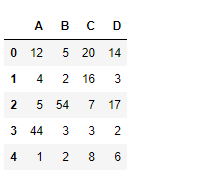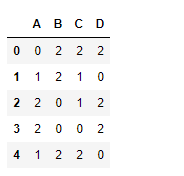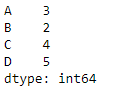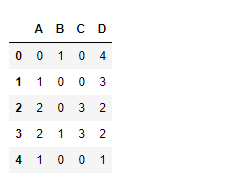Related Articles

# Python | Pandas dataframe.mod()

• Last Updated : 23 Jul, 2021

Python is a great language for doing data analysis, primarily because of the fantastic ecosystem of data-centric python packages. Pandas is one of those packages and makes importing and analyzing data much easier.
Pandas dataframe.mod() function returns modulo of dataframe and other, element-wise (binary operator mod). This function is essentially same as the Dataframe % other, but with support to substitute a fill_value for missing data in one of the inputs. This function can be used with either a series or a dataframe.

Syntax: DataFrame.mod(other, axis=’columns’, level=None, fill_value=None)
Parameters :
Other : Series, DataFrame, or constant
axis : For Series input, axis to match Series index on
level : Broadcast across a level, matching Index values on the passed MultiIndex level
fill_value : Fill existing missing (NaN) values, and any new element needed for successful DataFrame alignment, with this value before computation. If data in both corresponding DataFrame locations is missing the result will be missing
Returns : result : DataFrame

Example #1: Use mod() function to find the modulo of each value in the dataframe withe a constant.

## Python3

 `# importing pandas as pd``import` `pandas as pd` `# Creating the dataframe``df ``=` `pd.DataFrame({``"A"``:[``12``, ``4``, ``5``, ``44``, ``1``],``                   ``"B"``:[``5``, ``2``, ``54``, ``3``, ``2``],``                   ``"C"``:[``20``, ``16``, ``7``, ``3``, ``8``],``                   ``"D"``:[``14``, ``3``, ``17``, ``2``, ``6``]})` `# Print the dataframe``df`Lets use the dataframe.mod() function to find the modulo of dataframe with 3

## Python3

 `# find mod of dataframe values with 3``df.mod(``3``)`

Output :Example #2: Use mod() function to find the modulo with a series over the column axis.

## Python3

 `# importing pandas as pd``import` `pandas as pd` `# Creating the dataframe``df ``=` `pd.DataFrame({``"A"``:[``12``, ``4``, ``5``, ``44``, ``1``],``                   ``"B"``:[``5``, ``2``, ``54``, ``3``, ``2``],``                   ``"C"``:[``20``, ``16``, ``7``, ``3``, ``8``],``                   ``"D"``:[``14``, ``3``, ``17``, ``2``, ``6``]})` `# Print the dataframe``df`Let’s create the series object

## Python3

 `# create a series``sr ``=` `pd.Series([``3``, ``2``, ``4``, ``5``])` `# setting its column index similar to the dataframe``sr.index ``=``[``"A"``, ``"B"``, ``"C"``, ``"D"``]` `# print the series``sr`Lets use the dataframe.mod() function to find the modulo of dataframe with series

## Python3

 `# find mod of dataframe values with series``# axis = 1 indicates column axis``df.mod(sr, axis ``=` `1``)`

Output :Attention geek! Strengthen your foundations with the Python Programming Foundation Course and learn the basics.

To begin with, your interview preparations Enhance your Data Structures concepts with the Python DS Course. And to begin with your Machine Learning Journey, join the Machine Learning – Basic Level Course

My Personal Notes arrow_drop_up